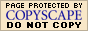Finding the Next Value in a Sequence:
Non-Mathematical "Sequences"
(page 6 of 7)

Sections: Common differences, Recursions, General examples, Non-math "sequences"There are quite a few sequences handed out to students in math classes that are not even mathematical sequences. Since this bugs me, I'll try to start a collection here of "spoilers", so you can short-circuit the process of frustration and find your answer here. For instance:

• Find the next number in the sequence:
31, 28, 31, 30, ...
• January has 31 days.
February has
28 days.
March has
31 days.
April has
30 days.   Copyright © Elizabeth Stapel 2002-2011 All Rights Reserved

Advertisement

• Find the next character in the sequence: J, F, M, A, ...
• "January" starts with "J".
"February" starts with "F".
"March" starts with "M".
"April" starts with "A".

• Find the next character in the sequence: S, M, T, W, ...
• "Sunday" starts with "S".
"Monday" starts with "M".
"Tuesday" starts with "T".
"Wednesday" starts with "W".

•  Find the next character in the sequence: Z, O, T, T, F, F, S, S,...
• "zero" starts with "Z"
"one" starts with "O"
"two" starts with "T"

• Find the next character in the sequence: A, E, F, H, ...
• "A" is written with all straight lines.
"B" is written with curvy and straight lines.
"C" is written with curvy lines.
"D" is written with curvy and straight lines.
"E" is written with all straight lines.
"F" is written with all straight lines.
"G" is written with curvy and straight lines.
"H" is written with all straight lines.

• Find the next character in the sequence: C, J, O, ...

This has nothing to do with math, and works by the same reasoning as the preceding example; it just takes the all-curvy-line letters, instead of the all-straight-line letters..

• Find the next number in the sequence: 3, 3, 5, 4, 4, ...
• "1" is spelled as "one", which has three letters.
"
2" is spelled as "two", which has three letters.
"
3" is spelled as "three", which has five letters.
"
4" is spelled as "four", which has four letters.
"
5" is spelled as "five", which has four letters.

Another of this sort is 3, 4, 4, 7, 10 (or 11), etc, which represents the numbers of letters (or characters in the squares of 1, 2, 3, 4, 5, etc; namely, 1, 4, 9, 16, 25, etc. (The difference will come from whether or not hyphens are included.)

• Find the next two numbers in the following sequence: 2, 3, 3, 5, 10, 13, 39,....

This one looks mathematical, and it is, sort of. But don't look for an overall pattern, because there isn't one. This one is:

2 + 1 = 3
3 × 1 = 3
3 + 2 = 5
5 × 2 = 10
10 + 3 = 13
13 × 3 = 3

So the next steps will be "plus 4" (so 39 + 4 = 43) and "times 4" (so 43 × 4 = 172). The next two numbes will be 43 and 172.

• Find the next two numbers in the sequence: 8, 5, 4, 9, 1,...

This one will be more obvious if I spell things out:

"8" is spelled as "eight", which starts with an "e".
"
5" is spelled as "five", which starts with an "f" and an "i".
"
4" is spelled as "four", which starts with an "f" and an "o".
"
9" is spelled as "nine", which starts with an "n".
"
1" is spelled as "one", which starts with an "o".

Golly, they're spelling the digit names and then listing the names alphabetically. So, hmm, "seven" and "six" would come next.

• Find the next number in the sequence: 7, 8, 5, 5, 3, 4, ...
• "January" is spelled with seven letters.
"February" is spelled with eight letters.
"March" is spelled with five letters.
"April" is spelled with five letters.
"May" is spelled with three letters.
"June" is spelled with four letters.

<< Previous  Top  |  1 | 2 | 3 | 4 | 5 | 6 | 7  |  Return to Index  Next >>

 Cite this article as: Stapel, Elizabeth. "Non-Mathematical 'Sequences' and Their Answers." Purplemath. Available from     https://www.purplemath.com/modules/nextnumb6.htm. Accessed [Date] [Month] 2016

MathHelp.com Courses
This lesson may be printed out for your personal use.Copyright © 2021  Elizabeth Stapel   |   About   |   Terms of Use   |   Linking   |   Site Licensing Contact Us